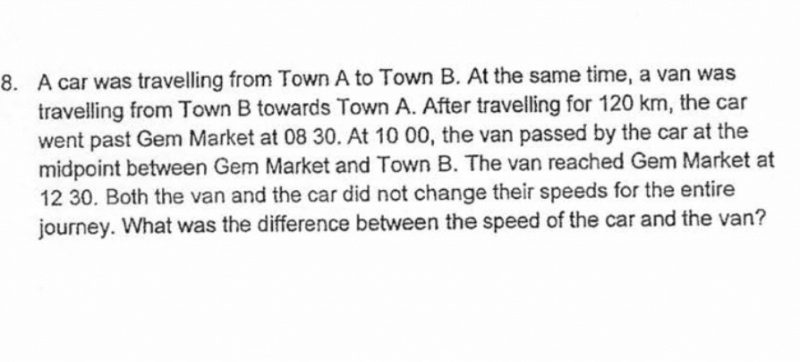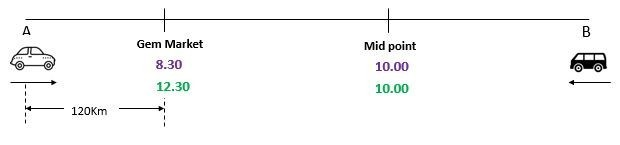Questionhi! can help us with this qn? tq!

Source: .

Do correct me if I am wrong. In my humble opinion, when two objects of different speed travel over the same distance without changing their speed, the difference in the amount of time taken will differ according to the distance travelled. However, the ratio in terms of time taken will remain the same.

Example 1

Speed of Car = 120 km/h

Speed of Van = 60 km/h

If both objects travels over 240km, the Car will take 2 hours and the Van will taken 4 hours. The difference in the amount of time taken will be 2 hours

If both objects travels over 360 km, the Car will take 3 hours and the Van will taken 6 hours. The difference in the amount of time taken will be 3 hours

As you can see in this example, the difference in terms of time taken is not the same when the distance travelled is different. Hence, we cannot conclude that the Van will take 1 hour more than the car to reach Town A from the Gem Market

Example 2

Speed of Car = 120 km/h

Speed of Van = 60 km/h

If both objects travels over 240km, the Car will take 2 hours and the Van will taken 4 hours. The time taken by the car to the time taken by the van in ratio will be 1 : 2 (Simplest form)

If both objects travels over 360, the Car will take 3 hours and the Van will taken 6 hours. The time taken by the car to the time taken by the van in ratio will also  be 1 : 2 (Simplest form)

As you can see in this example, the ratio in terms of time taken is the same even when the distance travelled is different

Let us apply this concept to the above question. The Car took 1.5 hours to travel from the Gem market to the mid point (0830 to 1000). Whereas the Van took 2.5 hours to travel from mid point to Gem market (1000 to 1230). Hence, the ratio in terms of time taken by the car to the time taken by the van over the same distance is 1.5 : 2.5. In its simplest form, it will be 3 : 5.

Since the ratio will maintain the same, we can conclude that the ratio in terms of time taken to travel 120 km will also be 3 : 5.

Therefore:

3 units = 1 hour

1 unit = 1/3 hour

5 units = 5/3 hour (Time taken by Van to travel 120 km)

120km ÷ 5/3 hour = 72 km/h (Speed of Van)

120 km/h – 72 km/h = 48 km/h (Difference in Speed between Car and Van)

2 Replies 0 Likes ✔Accepted Answer

thank u for the detailed explanation!

0 Replies 0 LikesTo travel from Gem Market to mid-point, Car takes 1 1/2 hours

In the other direction, to travel from mid-point to Gem Market, Van takes 2  1/2 hrs

Difference in travel duration is 1 hours and the van is slower than the car.

Time taken by car to cover distance between Gem Market and Town B = 3Hrs.

Time taken by van to cover distance between Gem Market and Town B = 5Hrs.

Ratio of time taken by car to van = 3 : 5

Since it is the middle point, time taken by van to travel between town B and mid-point will also be 2 1/2  hrs. So, Van would have started from town B at 7.30

Since both the car and van start at the same time, car should start from Town A at 7.30

For car,  duration to reach Gem Market = 1hr

Speed of car = 120/1   = 120 km/hr

Ratio of time taken, between car and van = 3: 5, so time taken by Van is 5/3 hr.

Speed of van = 120 x 3 /5  = 72 km/hr

Difference in speed = 120 – 72 = 48km/hr

1 Reply 0 Likes

Do correct me if I am wrong. In my humble opinion, when two objects of different speed travel over the same distance without changing their speed, the difference in the amount of time taken will differ according to the distance travelled. However, the ratio in terms of time taken will remain the same.

Example 1

Speed of Car = 120 km/h

Speed of Van = 60 km/h

If both objects travels over 240km, the Car will take 2 hours and the Van will taken 4 hours. The difference in the amount of time taken will be 2 hours

If both objects travels over 360 km, the Car will take 3 hours and the Van will taken 6 hours. The difference in the amount of time taken will be 3 hours

As you can see in this example, the difference in terms of time taken is not the same when the distance travelled is different. Hence, we cannot conclude that the Van will take 1 hour more than the car to reach Town A from the Gem Market

Example 2

Speed of Car = 120 km/h

Speed of Van = 60 km/h

If both objects travels over 240km, the Car will take 2 hours and the Van will taken 4 hours. The time taken by the car to the time taken by the van in ratio will be 1 : 2 (Simplest form)

If both objects travels over 360, the Car will take 3 hours and the Van will taken 6 hours. The time taken by the car to the time taken by the van in ratio will also  be 1 : 2 (Simplest form)

As you can see in this example, the ratio in terms of time taken is the same even when the distance travelled is different

Let us apply this concept to the above question. The Car took 1.5 hours to travel from the Gem market to the mid point (0830 to 1000). Whereas the Van took 2.5 hours to travel from mid point to Gem market (1000 to 1230). Hence, the ratio in terms of time taken by the car to the time taken by the van over the same distance is 1.5 : 2.5. In its simplest form, it will be 3 : 5.

Since the ratio will maintain the same, we can conclude that the ratio in terms of time taken to travel 120 km will also be 3 : 5.

Therefore:

3 units = 1 hour

1 unit = 1/3 hour

5 units = 5/3 hour (Time taken by Van to travel 120 km)

120km ÷ 5/3 hour = 72 km/h (Speed of Van)

120 km/h – 72 km/h = 48 km/h (Difference in Speed between Car and Van)

2 Replies 0 Likes ✔Accepted Answer

thank u for the detailed explanation!

0 Replies 0 Likes